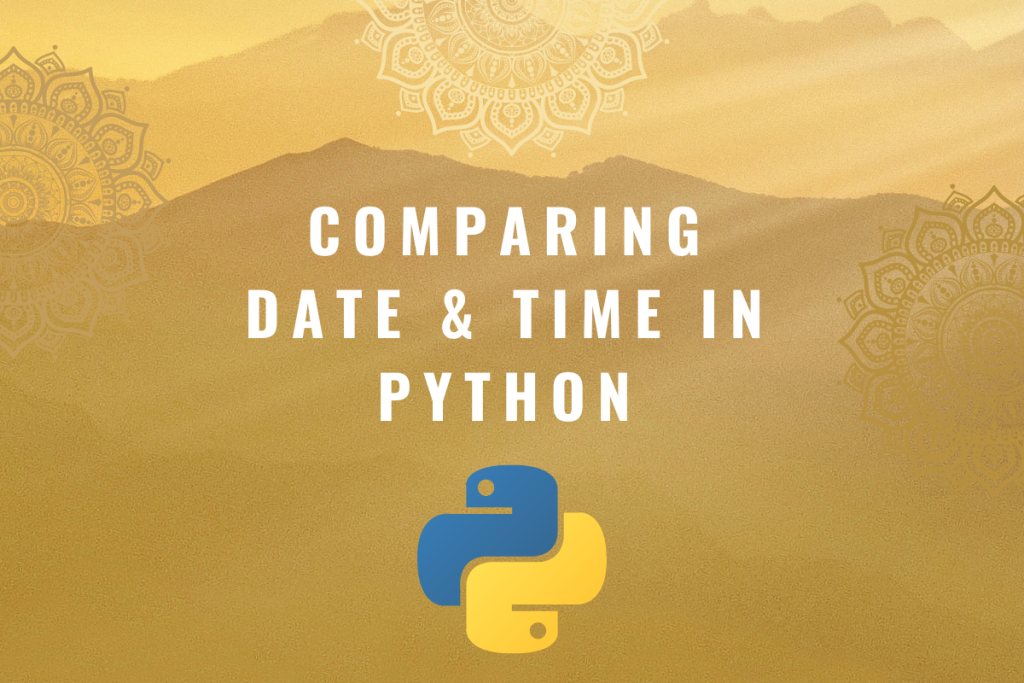# Comparing Date & Time in Python [Easy Step By Step]When one has to analyze data, it leaves no exception. The tool that is to be deployed for the analysis must be compatible with handling the various types of data that can be thrown at it. The data can be in the form of text, fractions, dates, integers, time & so. Python is one of the most sought tools to analyze data and has its own set of modules to deal with this data diversity.

This article shall focus on demonstrating the comparison between different dates & times using python. By different dates & times, the inference is towards the dates & times in different time zones.

Prima face the time may be the same, but when the time zone is factored in, it is a whole different picture. We shall set out to explore that by importing some of the libraries in python – pytz & datetime.

It can be done by typing the following code.

```import datetime
import pytz
```

## Comparing Dates in Python

The module datetime contains both date & time, so we shall only choose the date for comparison in this section. This can be done by extracting only the date from the above module using the below code.

```from datetime import date
```

Now let us try comparing two dates to determine whether they share the same day of the week. We shall first call today’s date using the following code.

```date.today()
```

What if one chooses to use datetime.today() instead of date.today()? This shall lead to the following result since we have already chosen only to use date from the datetime module.

Let us now move on with feeding another date for comparing it with the current date. But exercise caution while typing the entries for months or dates, since Python is not happy with leading zeros in these places. Else the following error shall appear.

So without the leading zeros, the code becomes,

```dt_1=datetime.datetime(2020,5,6)
```

Now that we know how to construct a date, let us now compare the two dates using the weekday() to see if they share the same weekday or not.

```if date.today().weekday()==date(2020,5,6).weekday():
print('Both dates share same weekday')
else:
print('Both dates do not share same weekday')
```

Executing the above code gives us the below output.

## Comparing Different Time Zones in Python

In this section, we shall try to compare the difference between the two time zones & verify whether they are the same. For this, we shall use an exclusive command in Python known as the timedelta(). Following is its syntax,

```timedelta(days=0, seconds=0, microseconds=0, milliseconds=0, minutes=0, hours=0, weeks=0)
```

This command cannot be used right away, rather be imported from the datetime library using,

```from datetime import timedelta
```

The difference in time between any two dates or times used is what timedelta helps in indicating. Let us set it to 3 hours, 30 minutes and assign it to a variable ‘Td’ to verify whether the difference between any two selected time zones matches with it.

```Td=timedelta(hours=3, minutes=30)
```

Now we shall include both time & date using the following code since we were only limited to the date in the previous section.

```from datetime import datetime
```

Once done, the current times of the Indian time zone & Australian time zone are assigned to the variables ‘tind’ and ‘taus’ respectively.

```tind=pytz.timezone('Asia/Kolkata')
taus=pytz.timezone('Australia/Brisbane')
```

Then using the if logic the comparison is done as follows.

```if Td == datetime.now(tind)-datetime.now(taus):
print('Difference in Time Zone = 3hr 30min')
else:
print('Difference in Time Zone != 3hr 30min')
```

The following is the output once the code is executed.